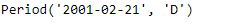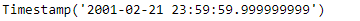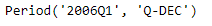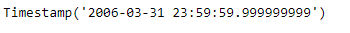Open in App
Not now

# Python | Pandas Period.end_time

• Last Updated : 06 Jan, 2019

Python is a great language for doing data analysis, primarily because of the fantastic ecosystem of data-centric python packages. Pandas is one of those packages and makes importing and analyzing data much easier.

Pandas` Period.end_time` attribute returns a Timestamp object containing the end time of the given period object.

Syntax : Period.end_time

Parameters : None

Return : Timestamp object

Example #1: Use `Period.end_time` attribute to find the end time for the given Period object.

 `# importing pandas as pd``import` `pandas as pd`` ` `# Create the Period object``prd ``=` `pd.Period(freq ``=``'D'``, year ``=` `2001``, month ``=` `2``, day ``=` `21``)`` ` `# Print the Period object``print``(prd)`

Output :Now we will use the `Period.end_time` attribute to find the end time for prd object.

 `# return the end time``prd.end_time`

Output :As we can see in the output, the `Period.end_time `attribute has returned a Timestamp object which contains the date value as well as the end time of the given period.

Example #2: Use `Period.end_time` attribute to find the end time for the given Period object.

 `# importing pandas as pd``import` `pandas as pd`` ` `# Create the Period object``prd ``=` `pd.Period(freq ``=``'Q'``, year ``=` `2006``, quarter ``=` `1``)`` ` `# Print the object``print``(prd)`

Output :Now we will use the `Period.end_time` attribute to find the end time for prd object.

 `# return the end time``prd.end_time`

Output :As we can see in the output, the `Period.end_time `attribute has returned a Timestamp object which contains the date value as well as the end time of the given period object.

My Personal Notes arrow_drop_up